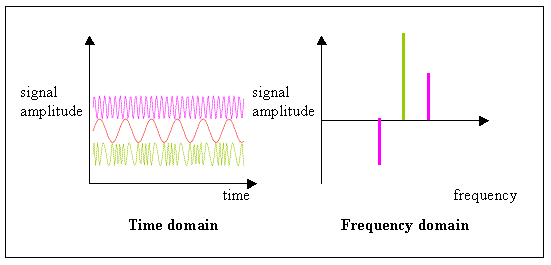# Difference between time domain and frequency domain

### There is the difference between time domain and frequency domain are given below,

Time domain Frequency domain
The advantage is that, they are relatively fast and requires less memory than inverting the Helmholtz operator. The advantage is that the frequency domain allows for techniques which could be used to determine the stability of the system.
A time domain graph shows how a signal changes over time. The frequency domain graph shows how much of the signal lies within each given frequency band over a range of frequencies.
Time domain is the domain for analysis of mathematical functions or signals with respect to time. Frequency domain is the domain for analysis of mathematical functions or signals with respect to frequency.
The time domain systems tend to use photon counting detectors which are slow but highly sensitive. The frequency domain systems are relatively inexpensive, easy to develop and use and can provide very fast temporal sampling.